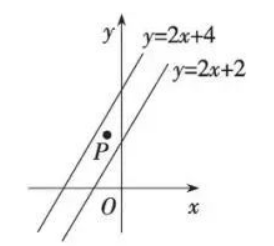$\text{A.}$ $2 < a < 4$ $\text{B.}$ $1 < a < 3$ $\text{C.}$ $1 < a < 2$ $\text{D.}$ $0 < a < 2$